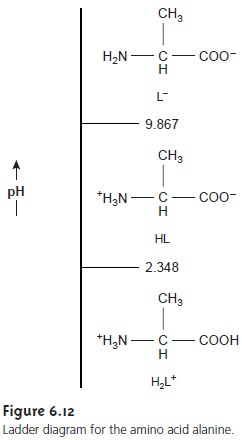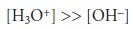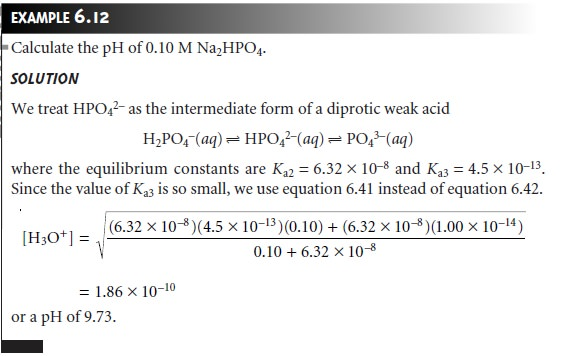Home | | Modern Analytical Chemistry | Solving Equilibrium Problems: pH of a Polyprotic Acid or Base

# Solving Equilibrium Problems: pH of a Polyprotic Acid or Base

A more challenging problem is to find the pH of a solution prepared from a polyprotic acid or one of its conjugate species.

pH of a Polyprotic Acid or Base

A more challenging problem is to find the pH of a solution prepared from a polyprotic acid or one of its conjugate species. As an example, we will use the amino acid alanine whose structure and acid dissociation constants are shown in Figure 6.11.## pH of 0.10 M H2L+

Alanine hydrochloride is a salt consisting of the diprotic weak acid H2L+ and Clâ€“. Because H2L+ has two acid dissociation reactions, a complete systematic solution to this problem will be more complicated than that for a mono- protic weak acid. Using a ladder diagram (Figure 6.12) can help us simplify the problem. Since the areas of predominance for H2L+ and Lâ€“ are widely separated, we can assume that any solution containing an appreciable quantity of H2L+ will con- tain essentially no Lâ€“. In this case, HL  is such a weak acid that H2L+ behaves as if it were a monoprotic weak acid.To find the pH of 0.10 M H2L+, we assume thatBecause H2L+ is a relatively strong weak acid, we cannot simplify the problem fur- ther, leaving us withSolving the resulting quadratic equation gives the [H3O+] as 1.91 x 10â€“2 M or a pH of 1.72. Our assumption that [H3O+] is significantly greater than [OHâ€“] is acceptable.

## pH of 0.10 M Lâ€“

The alaninate ion is a diprotic weak base, but using the ladder dia- gram as a guide shows us that we can treat it as if it were a monoprotic weak base. Following the steps in Example 6.11 (which is left as an exercise), we find that the pH of 0.10 M alaninate is 11.42.## pH of 0.1 M HL

Finding the pH of a solution of alanine is more complicated than that for H2L+ or Lâ€“ because we must consider two equilibrium reactions involving HL. Alanine is an amphiprotic species, behaving as an acid

HL(aq)+ H2O(l) < = = = = > H3O+(aq)+ Lâ€“(aq)

and a base

HL(aq)+ H2O(l)  < ===   ==   >  OHâ€“(aq)+ H2L+(aq)

As always, we must also consider the dissociation of water

2H2O(l)     < == == >   H3O+(aq)+ OHâ€“(aq)

This leaves us with five unknowns ([H2L+], [HL], [Lâ€“], [H3O+], and [OHâ€“]), for which we need five equations. These equations are Ka2 and Kb2 for HL,From the ladder diagram it appears that we may safely assume that the concentra- tions of H2L+ and Lâ€“ are significantly smaller than that for HL, allowing us to sim- plify the mass balance equation to## Triprotic Acids and Bases, and Beyond

The treatment of a diprotic acid or base is easily extended to acids and bases having three or more acidâ€“base sites. For a tripro- tic weak acid such as H3PO4, for example, we can treat H3PO4 as if it was a mono- protic weak acid, H2PO4â€“ and HPO 2â€“ as if they were intermediate forms of diprotic weak acids, and PO 3â€“ as if it was a monoprotic weak base.Study Material, Lecturing Notes, Assignment, Reference, Wiki description explanation, brief detail
Modern Analytical Chemistry: Equilibrium Chemistry : Solving Equilibrium Problems: pH of a Polyprotic Acid or Base |